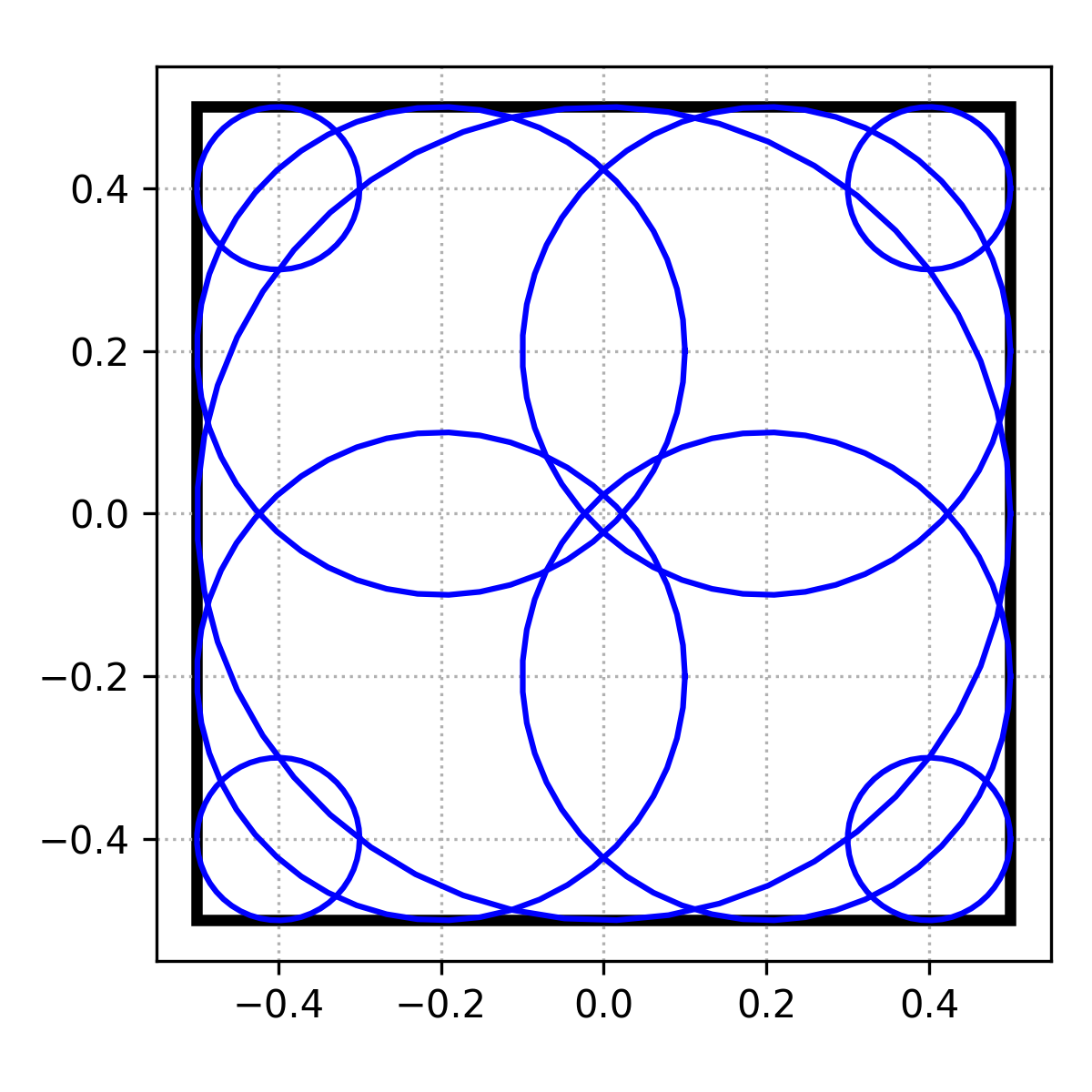# microstructpy.geometry.Square¶

class microstructpy.geometry.Square(**kwargs)[source]

A square.

This class contains a generic, 2D square. It is derived from the microstructpy.geometry.Rectangle class and contains the side_length property, rather than multiple side lengths.

Parameters
• side_length (float) – (optional) Side length. Defaults to 1.

• center (list) – (optional) Center of rectangle. Defaults to (0, 0).

• corner (list) – (optional) Bottom-left corner.

approximate(x1=None)[source]

Approximate square with a set of circles

This method approximates a square with a set of circles. These circles are spaced uniformly along the edges of the square with distance x1 between them.

Example

For a square with side_length=1, and x1=0.2, the approximation would look like Fig. 30.Fig. 30 Circular approximation of square.¶
Parameters

x1 (float or None) – (optional) Spacing between the circles. If not specified, the spacing is 0.25x the side length.

Returns

An Nx3 array, where each row is a circle and the columns are x, y, and r.

Return type

numpy.ndarray

classmethod area_expectation(**kwargs)[source]

Expected area of square

This method computes the expected area of a square with distributed side length. The expectation is:

$\mathbb{E}[A] = \mathbb{E}[S^2] = \mu_S^2 + \sigma_S^2$

Example

>>> import scipy.stats
>>> import microstructpy as msp
>>> S = scipy.stats.expon(scale=2)
>>> S.mean()^2 + S.var()
8.0
>>> msp.geometry.Square.area_expectation(side_length=S)
8.0

Parameters

**kwargs – Keyword arguments, same as Square but the inputs can be from the scipy.stats module.

Returns

Expected/average area of the square.

Return type

float

best_fit(points)

Find rectangle of best fit for points

Parameters

points (list) – List of points to fit.

Returns

an instance of the class that best fits the points.

Return type

Rectangle

plot(**kwargs)

Plot the rectangle.

This function adds a matplotlib.patches.Rectangle patch to the current axes. The keyword arguments are passed through to the patch.

Parameters

**kwargs (dict) – Keyword arguments for the patch.

within(points)

Test if points are within n-box.

This function tests whether a point or set of points are within the n-box. For the set of points, a list of booleans is returned to indicate which points are within the n-box.

Parameters

points (list or numpy.ndarray) – Point or list of points.

Returns

Flags set to True for points in geometry.

Return type
property angle

Rotation angle of rectangle - degrees

Type

float

property angle_deg

Rotation angle of rectangle - degrees

Type

float

Rotation angle of rectangle - radians

Type

float

property area

Area of rectangle

Type

float

property bounds

(lower, upper) bounds of the box

Type

list

property corner

bottom-left corner

Type

list

property length

Length of rectangle, side length along 1st axis

Type

float

property limits

(lower, upper) bounds of the box

Type

list

property n_dim

Number of dimensions, 2

Type

int

property n_vol

area, volume of n-box

Type

float

property sample_limits

(lower, upper) bounds of the sampling region of the box

Type

list

property side_length

length of the side of the square.

Type

float

property width

Width of rectangle, side length along 2nd axis

Type

float